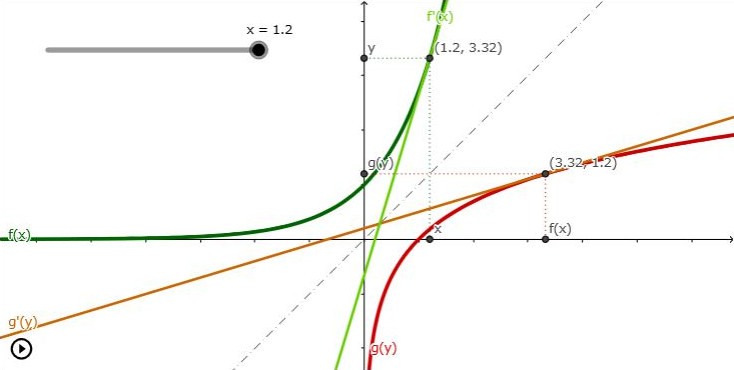# Visualizing the inverse theorem$$\newcommand{annotation}{ {~~~~~~\raise 0.8em {\color{grey}\scriptsize{ #1 }}} } \newcommand{Annotation}{ \rlap{~~~~~~\raise 0.8em {\color{grey}\scriptsize{ #1 }}} } \newcommand{d}{ \text{d} }$$

## The gist

The inverse function theorem is a an elegant observation stemming from the symmetry of functions and their inverse along the $$y=x$$ axis.

Consider a function $$y = f(x)$$ and its inverse $$x = g(y)$$, the inverse theorem states that you can compute $$g'(y)$$ purely based on $$f'$$ without prior knowledge of $$g$$ (notice, but temporarly ignore that the equality below links two seemingly unrelated variables):

$$g'({\color{red} y }) = \frac{1}{f'({\color{green} x })}$$

This is a pretty neat fact, it means when you can't find the inverse $$g$$ you can still easily deduce its tangent $$g'$$ from the original function $$f$$.

## Geometric interpretation

The crux is that the inverse of the tangent $$g'$$ is the mirrored version of $$f'$$, which allows us to say $$g' = 1 / f'$$. So in practice, let's set x to some value and compute the tangent $$f'$$ at some point $$(x, \ y = f(x))$$. The the inverse theorem then tells us, $$g' = 1/f'$$, however, where do you think this tangent should lie $$(\cdots, \cdots)$$ ? Certainly not at $$(x, \ y)$$! Well, it actually goes through the mirrored point $$(y, \ x)$$. Just switching coordinates gives you the location of the inverse tangent $$g'$$. What's more is that just setting $$x$$ allows us to know $$y = f(x)$$, nowhere did we need $$g$$. This can be summarized by the equality below:

$$g'(f(x)) = \frac{1}{f'(x)}$$

Let's rephrase the above to help things sink in. We want to find the tangent of $$f$$ and $$g$$ but only given a variable $$x$$. So far we know that $$g'({\color{red} y }) = {1} / {f'({\color{green} x })}$$ but how do we deduce the right $${\color{red} y }$$ that corresponds to the tangent $$g'$$ of the inverse $$g$$? Well this is given by $$y = f(x)$$.

## Deriving the inverse theorem

Using the chain rule and the fact that $$g(f(x)) = x$$ it's simple to derive:

\begin{aligned} g\big ( { \color{darkblue} f(x)} \big ) & = x \\ \Rightarrow \Big [ g\big ({ \color{darkblue} f(x)}\big) \Big ]' & = \Big [ x \Big ]'\\ g'\big ({ \color{darkblue} f(x)}\big) . f'(x) & = 1 \\ g'\big ({ \color{darkblue} f(x)}\big) & = \frac{1}{f'(x)} \\ \end{aligned}

## Variation

By definition of the inverse $$f$$ and $$g$$ can be swapped:

\begin{aligned} f\big (g(x) \big ) & = x \\ \Rightarrow f'\big ({ g(y)}\big) &= \frac{1}{g'(y)} \end{aligned}

## Leibniz tricks

This one is neat, when using Leibniz notation to designate derivatives, it is very intuitive to get back the inverse theorem:

$$\frac{ \d x}{\d {\color{red} y}} = \frac{1}{{\d y}\over{\d {\color{green} x}}} \Annotation{ \text{ thinking in terms rate change between variables: } \\ x = g(y) \text{ and } y = f(y)}$$

We can see the above trick as renaming $$f:y$$ and $$g:x$$ :

\begin{aligned} \frac{ \d g}{\d y} \quad & = \quad \frac{1}{{\d f}\over{ \d x}}\\ \frac{ \d \big( g(y) \big) }{\d y} & = \frac{1}{{\d\big(f(x)\big)}\over{\d x}} \\ g'(y) \quad & = \quad \frac{1}{ f'(x) } \\ \end{aligned}

Notice also the notation is a tad abusive because the equality only holds because we have hidden information:

$\frac{ \d x}{\d {\color{red} y}} = \frac{ \d x(\text{...}) }{\d {\color{red} y}} = \frac{ \d x( y ) }{\d {\color{red} y}} = \frac{ \d x( {\color{red} y} = f({\color{green} x}) ) }{\d {\color{red} y}} = g'( f({\color{green} x})) = \frac{1}{{\d y}\over{\d {\color{green} x}}} = \frac{1}{ f'({\color{green} x}) }$

Consider $$h_i: \mathbb R \rightarrow \mathbb R$$; when making use of the Leibniz trick, keep in mind this change the input variable you use to evaluate the expression:

$$h_1({\color{red} y}) = \frac{ \d x}{\d {\color{red} y}}$$ $$h_2({\color{green} x}) = \frac{1}{{\d y}\over{\d {\color{green} x}}}$$

But that might turn out ok since we can always find a $$y$$ according to an $$x$$ with the original function $$y = f(x)$$:

$$h_1\big (f({\color{green} x}) \big) = h_2({\color{green} x}) = \frac{1}{{\d y}\over{\d {\color{green} x}}}$$ $$h_2\big (g({\color{red} y}) \big) = h_1({\color{red} y}) = \frac{ \d x}{\d {\color{red} y}}$$

# Proper theorem

This is the exact definition of the inverse theorem.

If $$f$$ is differentiable at $$c$$, $$f'$$ is continuous at $$c$$, and $$f'(c) \neq 0$$, there is an interval $$I$$ containing $$c$$ on which $$f^{-1}$$ exists and is differentiable. Moreover:

$${f^{\scriptscriptstyle -1}}^{\displaystyle'}\big (f(c) \big) = \frac{1} {f^\prime} (c)}$$

# Example

Consider:

$$f(x) = e^x + x^3 + x$$

The inverse $$f^{-1}$$ does not have a nice closed form expression but we can still compute $$f'^{-1}$$ if we set $$c$$; for instance $$c= 0$$

- $$f(0) = 1$$
- $$f'(x) = e^x + 3 x^2 + 1$$
- $$f'(0) = 1 + 1 = 2$$

$${f^{\scriptscriptstyle -1}}^{\displaystyle'}\big(f(0)\big) = {f^{\scriptscriptstyle -1}}^{\displaystyle'}(1) = \frac{1} {f^\prime} (0)} = \frac{1} {2}$$

The crux really lies in the fact we evaluate $${f^{\scriptscriptstyle -1}}^{\displaystyle'}(1)$$ at 1 and not at 0.

# Images